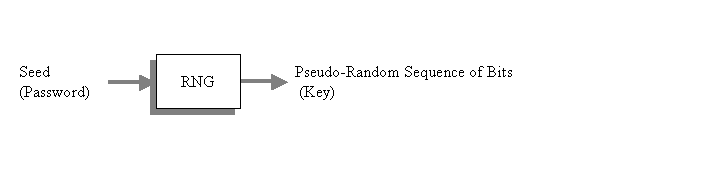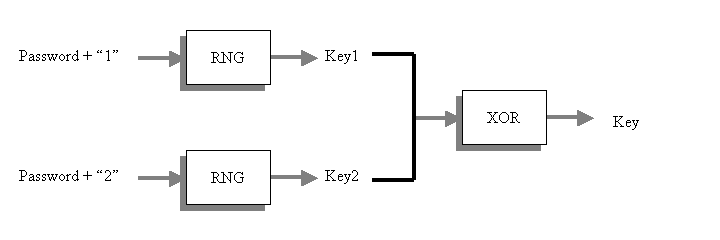# Key derivation using random number generators

Martin McBride, 2017-04-09
Tags cryptography key derivation random numbers
Categories cryptography

A psuedo-random number generator (RNG) is an algorithm which generates a stream of bits which have highly random statistical properties. The algorithm accepts a seed, in the form of an arbitrary length byte string. Any particular seed will always generate the same bit sequence, but different seeds will generate different sequences.

The application of RNG to key derivation is quite simple. The password is used as the seed value, and the bit sequence produced by the algorithm is used as the key. The advantage of this technique over hashing is that the key can be arbitrarily large (you can just keep on reading bits from the sequence).## The search space problem

The internal operation of many RNG algorithms acts to seriously restrict the search space.

What happens in some algorithms is that the initial seed is effectively hashed to quite a short length, eg 64 bits, before it is used to create the random sequence. This limitation usually arises, unavoidably, out of the structure of the algorithm.

What this means is that the RNG will accept a very long password, and produce a very long key, but the small search space means that you will not be getting the level of security you are expecting. For example, you can create a 1024 bit key, but the algorithm will only produce 264 different 1024 bit keys, whatever password you supply.

## Solving the problem

A solution to this problem is specified in PKCS#5. We describe a simplified version here.Here is the scheme. We take the original password, append the string "1" to the end of it, and use that string to seed the RNG. We use this to generate Key1, of the required bitlength (eg 1024 bits).

Then, we take the original password, append the string "2" to the end of it, and generate Key2 in a similar way.

Finally, we XOR the 2 values together to create the final key - this key has a much larger search space.

How does this work? We know that Key1 must belong to the 264 possible keys which make up the RNG search space. We also know that Key2 belongs to the same search space. However, Key1 and Key2 are both, in effect, random bit streams, then when you XOR the two together you will get a thirs random bit stream which almost certainly does not belong to the same search space.